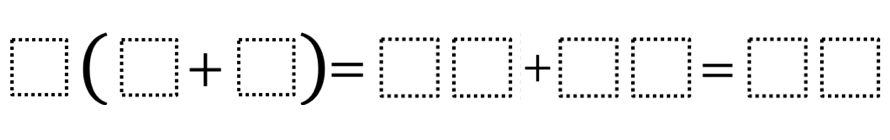Home > Grade 6 > Expressions & Equations > Distributive Property 3

# Distributive Property 3

Directions: Fill in the boxes below using all the digits 0 through 9 at most one time each to make the statement true.### Hint

Could one of the numbers in the first space be 1? 5? Why or why not?

Are there any limits on the size of the sum in parentheses?

3(6 + 9) = 18 + 27 = 45
6(3 + 9) = 18 + 54 = 72
9(3 + 6) = 27 + 54 = 81

Source: Julie Wright

## Subtracting Multi-Decimals

Directions: Use the digits 1 to 9, at most one time each, to fill in …

1.Steven Krolikowski

Julia,

Great task! I like that there are multiple solutions.

2.Because you showed the solution (a 2-digit number) as two boxes, I would use two boxes for the addends in the middle. Because I need more coffee, I didn’t “understand” that I needed to use ALL the digits 0 – 9 and since the solution was shown with two boxes for a two digit number, I assumed that each box could only hold one digit. Just a suggestion.

•Yes, you need to have a box for each digit.

3.I like this activity, but I agree with Mindy that the single box appears to indicate a single digit. Also, the instructions say to use each digit “at most” one time.

One solution for the single digit per blank option is:

2(3+5)=7+9=16

•While I agree this is a correct solution, the terms in the middle space don’t respectively reflect the terms of the bracket expansion. This adds a further confusion to Mindy’s point.

•Crystal Spellmann

Wow, Lauren, I like your thinking. I do agree with Monier about “the terms in the middle space don’t respectively reflect the terms of the bracket expansion”, but what might be an interesting problem to reflect your idea would be if the original problem had been #(#+#) = ## = #+#.

4.Hi – 2 of my students worked for a while on this and reached total frustration. To help them, I checked the answer, to see if I could offer guidance. It was only then that we realized that the single boxes sometimes represent two digits and sometimes represent one digit!! AAARGH!! And now I see that people have commented on that previously. Should have read the comments. Can you revise this one so that it is consistently 1 box – 1 digit? Thanks! And, yeah, we didn’t get that we needed to use all the digits. Otherwise, awesome!

5.9(5+3)=64+08=72

6.Michael Auciello

2 (8 + 0) = 9 + 7 = 16

7.8.9.